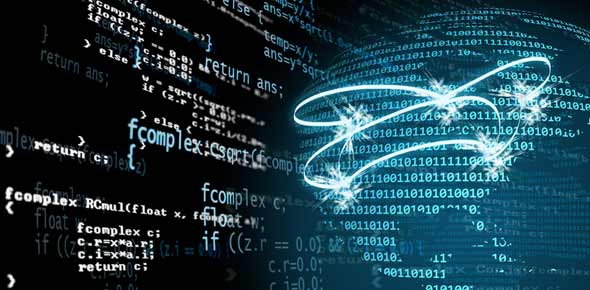25 Questions | Total Attempts: 613SettingsRelated Topics
• 1.
• A.

Calculating numbers

• B.

Grammar checking

• C.

• D.

Searching and retrieving

• 2.
The contents in cells A1 through A6 are 6, 3, 4, 7, 9, and 2. What function would return the answer 31?
• A.

=MAX(A1:A6)

• B.

=MIN(A1:A6)

• C.

=ROUND(A1:A6)

• D.

=SUM(A1:A6)

• 3.
When keying a formula in a spreadsheet, what must you key to inform the program that you are entering a formula instead of entering text?
• A.

• B.

Dovision operator

• C.

Equal sign

• D.

Substraction sign

• 4.
The intersection of a column and row in a spreadsheet is called what?
• A.

Cell

• B.

Column

• C.

Function

• D.

Row

• 5.
What is the horizontal portion of a spreadsheet that is identified by a number?
• A.

Cell

• B.

Column

• C.

Reference

• D.

Row

• 6.
A mathematical operation that is built into a spreadsheet program to perform shortcut calculations is called what?
• A.

Cell

• B.

Function

• C.

Label

• D.

Value

• 7.
Numerical data entered into a spreadsheet is called what?
• A.

Formula

• B.

Label

• C.

Text

• D.

Value

• 8.
What is alphabetical text entered into a spreadsheet?
• A.

Formula

• B.

Label

• C.

Text

• D.

Value

• 9.
What is a statement that performs a calculation in a spreadsheet?
• A.

Cell

• B.

Formula

• C.

Label

• D.

Value

• 10.
The cell in which you are currently working is known as what?
• A.

Active cell

• B.

• C.

Cell reference

• D.

• 11.
What is the vertical portion of a spreadsheet that is identified by a letter?
• A.

Cell

• B.

Column

• C.

Reference

• D.

Row

• 12.
Which function would be used to indicate the smallest value in a range of spreadsheet cells?
• A.

COUNT

• B.

MAX

• C.

MIN

• D.

SUM

• 13.
Which function would return the same answer as the formula =B2+B3+B4+B5+B6+B7?
• A.

=SUM(B2/B7)

• B.

=SUM(B2+B7)

• C.

=SUM(B2:B7)

• D.

=SUM(B2*B7)

• 14.
Cells that automatically change when copied are called what?
• A.

Absolute

• B.

Automation

• C.

Relational

• D.

Relative

• 15.
Repeated pound signs in a spreadsheet cell indicate what?
• A.

Column width too narrow

• B.

Column width too wide

• C.

Row height too narrow

• D.

Row height too wide

• 16.
What are cells that need to remain the same when copied?
• A.

Absolute

• B.

Automation

• C.

Relational

• D.

Relative

• 17.
The contents in cells A1 through A6 are 6, 3, 4, 7, 9, and 7. What function would return the answer 6?
• A.

=MAX(A1:A6)

• B.

=MIN(A1:A6)

• C.

=AVERAGE(A1:A6)

• D.

=SUM(A1:A6)

• 18.
The contents in cells A1 through A6 are 6, 3, 4, 7, 9, and 2.  What function would return the answer 2?
• A.

=MAX(A1:A6)

• B.

=MIN(A1:A6)

• C.

=ROUND(A1:A6)

• D.

=SUM(A1:A6)

• 19.
What are the three most common types of charts used to illustrate spreadsheet data?
• A.

Bar, line, and pie

• B.

Bar, line, and scattergram

• C.

Line, pie, and area

• D.

Pie, scattergram, and stacked bar

• 20.
• A.

• B.

• C.

Checks for inaccurate data

• D.

Writes formulas automatically

• 21.
What specific format would change 29.99 to \$29.99?
• A.

Currency

• B.

Number

• C.

Percentage

• D.

Scientific

• 22.
What are bar charts MOST effectively used to show?
• A.

Compare groups of similar data

• B.

Present scientific data

• C.

Show data as part of a whole

• D.

Show changes over a period of time

• 23.
A series of dialog boxes used to provide steps in creating a chart is called what?
• A.

Chart helper

• B.

Chart wizard

• C.

Guide master

• D.

Wizard maker

• 24.
What is the first step in creating a chart or graph?
• A.

Choose page setup for orientation

• B.

Choose the chart wizard for help

• C.

Select print preview to see graph

• D.

Select the data you wish to plot

• 25.
How is spreadsheet software used for personal use?
• A.

Doing language art homework

• B.